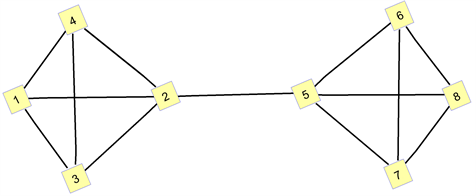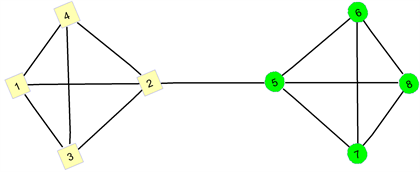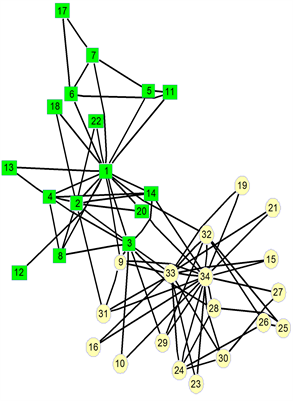# 基于节点重要度的社团划分方法研究Research on Community Division Method Based on Node Importance

• 全文下载: PDF(1012KB)    PP.1079-1087   DOI: 10.12677/AAM.2019.86124
• 下载量: 232  浏览量: 411   国家自然科学基金支持

By dividing the community structure existing in the complex network, it is helpful to discover the function, structure, hidden law and influence of the network. In order to get a better classification result of network community structure, and more ways of community division, this paper defines the node importance evaluation function of the network, and proposes a community partitioning algorithm based on node importance degree. The example shows that the algorithm can well clas-sify the community structure in complex networks.

1. 引言

2. 基础理论及相关概念

${a}_{ij}=\left\{\begin{array}{l}1,\left({v}_{i},{v}_{j}\right)\in E\\ 0,\left({v}_{i},{v}_{j}\right)\notin E\end{array}$

${C}_{d}\left({v}_{i}\right)={k}_{i}$

${C}_{D}\left({v}_{i}\right)=\frac{{k}_{i}}{\left(N-1\right)}$

$\stackrel{˜}{L}=D-A$

$\stackrel{˜}{L}={\left({l}_{ij}\right)}_{N×N}$$\stackrel{˜}{L}$ 的对角线上的元素 ${l}_{ii}$ 是节点 ${v}_{i}$ 的度，其他非对角线上的元素 ${l}_{ij}$ 则表示节点 ${v}_{i}$ 和节点 ${v}_{j}$

3. 基于节点重要度的社团划分方法

$F={C}_{D}\left({v}_{i}\right)-{e}_{ij}$

${e}_{ij}=\left\{\begin{array}{l}\frac{1}{{d}_{ij}},i\ne j\\ 0,i=j\end{array}$

${e}_{ij}$ 表示节点 ${v}_{i}$ 对节点 ${v}_{j}$ 的重要度影响力； ${d}_{ij}$ 表示网络中节点 ${v}_{i}$ 到节点 ${v}_{j}$ 的最短距离。

1) 输入具有n个节点的复杂网络 $G=〈V,E〉$。写出网络的邻接矩阵并将邻接矩阵初始化，输入邻接矩阵的上角矩阵；

2) 计算节点 ${v}_{i}$ 的自身的度指标 ${C}_{D}\left({v}_{i}\right)=\frac{{k}_{i}}{\left(N-1\right)}$ ；随后计算节点 ${v}_{j}$ 对节点 ${v}_{i}$ 的重要度影响值 ${e}_{ij}=\left\{\begin{array}{l}\frac{1}{{d}_{ij}},i\ne j\\ 0,i=j\end{array}$ ；最后输出节点重要度评价函数 $F={C}_{D}\left({v}_{i}\right)-{e}_{ij}$ 所形成的矩阵；

3) 求此矩阵的最小的两个特征值及特征值所对应的特征向量，根据元素的正负便可将网络划分成两个社团。特征向量中所有的正的元素对应的网络节点所形成的属于同一个社团，其他负的元素对应的节点属于第二个社团；

4) 根据以上步骤，可以将网络进行划分，刷新图形便可得出划分后的图形。Figure 1. 8 points of undirected networkTable 1. Degree indicators of the corresponding nodesTable 2. Feature vectors for corresponding nodesTable 3. Importance evaluation function values for corresponding nodesFigure 2. Results of the division of 8 points of undirected networks

4. 实验结果与分析

Zachary空手道俱乐部网络是复杂网络  等相关领域的典型测试网络的数据集。在20世纪70年代初期，社会学家Zachary耗时两年的时间来研究美国一所大学中空手道俱乐部里34名成员之间的社会关系的网络，基于这些成员在俱乐部内外部的交往情况，构造了成员之间的社会网络体系。此网络包含34个节点和78条边，节点代表俱乐部的成员，两个节点之间有一条边则意味着相应的两个成员间至少是有交往关系的朋友。他通过观察发现，是因为俱乐部中的主管JohnA (node1)和教练员Mr. Hi (node34)之间因为是否提高俱乐部收费问题产生了一些矛盾和分歧，因此俱乐部便被分裂成分别以主管和教练为代表的两个团体。Figure 3. Karate club network membership networkTable 4. Degree indicators of each node of the karate club networkTable 5. Feature vectors of corresponding nodes in karate club networkTable 6. Division of each node of the karate club networkFigure 4. The results of the algorithm’s division of the karate club networkFigure 5. Results of the division of the karate club network by spectral equalization

5. 结束语

NOTES

*通讯作者。

  孙玺菁, 司守奎. 复杂网络算法与应用[M]. 北京: 国防工业出版社, 2015.  汪小帆, 李翔, 陈关荣. 复杂网络理论及其应用[M]. 北京: 清华大学出版社, 2006.  Pothen, A., Simon, H. and Liou, K.-P. (1990) Partitioning Sparse Matrices with Eigenvectors of Graphs. SIAM Journal on Matrix Analysis and Applications, 11, 430. https://doi.org/10.1137/0611030  Scott, J. (2001) Social Network Analysis: A Handbook. 2nd Edition, Sage Publications, London.  Fiedler, M. (1973) Algebraic Connectivity of Graphs. Czechoslovak Mathematical Journal, 23, 298-305.  Unser, M., Aldroubi, A. and Eden, M. (1993) B-Spline Signal Processing: Part II Efficient Design and Applications. IEEE Transactions on Signal Processing, 41, 834-848. https://doi.org/10.1109/78.193221  吴建平, 宋君强, 张卫民, 等. 计算Fiedler向量的一种高效准确方法[J]. 计算机学报, 2013(11): 2266-2273.  吴卫江, 周静, 李国和. 一种基于节点重要度的社团划分算法[J]. 中南民族大学学报, 2016(1) :0119-04.  周漩, 张凤鸣, 李克武, 等. 利用重要度评价矩阵确定复杂网络关键节点[J]. 物理学报, 2012(5): 1-7.  Zachary, W.W. (1977) An Information Flow Model for Conflict and Fission in Small Groups. Journal of Anthropological Re-search, 33, 452-473. https://doi.org/10.1086/jar.33.4.3629752